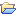# Uncertainty

This category contains 5 nodes.

##### Entropy Uncertainty ScorerStreamable

Calculates the entropy uncertainty score of a class probability distribution.

##### Least Confident Uncertainty ScorerStreamable

Calculates the least confident uncertainty score of a class probability distribution.

##### Margin Uncertainty ScorerStreamable

Calculates the margin uncertainty score for a class probability distribution.

##### Variance Uncertainty ScorerStreamable

Calculates the variance uncertainty score of a class probability distribution.

##### Variance Uncertainty ScorerStreamableDeprecated

Calculates the variance of a class probability distribution.